Win up to 100% scholarship on Aakash BYJU'S JEE/NEET courses with ABNAT Win up to 100% scholarship on Aakash BYJU'S JEE/NEET courses with ABNAT

# JEE Main 2022 July 28 – Shift 1 Chemistry Question Paper with Solutions

The JEE Main 2022 July 28 – Shift 1 Chemistry Question Paper with Solutions is given on this page. JEE 2022 question papers are the best tools for JEE Main preparation. Practising these question papers will strengthen your knowledge and helps to find out the topics which you need more learning. JEE Main 2022 solutions are well explained so that you can easily understand each and every step in the questions. Significant practice of the JEE Mains 2022 question papers will help attain outstanding results. Learn the JEE Main 2022 July 28 Chemistry Shift 1 Question Paper with Solutions given below.

## JEE Main 2022 28th July Shift 1 Chemistry Question Paper and Solutions

SECTION – A

Multiple Choice Questions: This section contains 20 multiple choice questions. Each question has 4 choices (1), (2), (3) and (4), out of which ONLY ONE is correct.

1. Identify the incorrect statement from the following.

(A) A circular path around the nucleus in which an electron moves is proposed as Bohr’s orbit.

(B) An orbital is the one electron wave function (Ψ) in an atom.

(C) The existence of Bohr’s orbits is supported by hydrogen spectrum.

(D) Atomic orbital is characterised by the quantum numbers n and l only.

Sol. Atomic orbital is characterised by the quantum numbers n, l and m.

Hence option D is incorrect.

2. Which of the following relation is not correct?

(A) ΔH = ΔU – PΔV

(B) ΔU = q + W

(C) ΔSsys + ΔSsurr ≥ 0

(D) ΔG = ΔH – TΔS

Sol. ΔH = ΔU + PΔV

Hence option A is incorrect.

3. Match List-I with List-II :

List-I List-II
(A) Cd(s) + 2Ni(OH)3(s) → CdO(s) + 2Ni(OH)2(s) + H2O(l) (I) Primary battery
(B) Zn(Hg) + HgO(s) → ZnO(s) + Hg(l) (II) Discharging of secondary battery
(C) 2PbSO4(s) + 2H2O(l) → Pb(s) + PbO2(s) + 2H2SO4(aq) (III) Fuel cell
(D) 2H2(g) + O2(g) → 2H2O(l) (IV) Charging of secondary battery

Choose the correct answer from the options given below:

(A) (A)-(I), (B)-(II), (C)-(III), (D)-(IV)

(B) (A)-(IV), (B)-(I), (C)-(II), (D)-(III)

(C) (A)-(II), (B)-(I), (C)-(IV), (D)-(III)

(D) (A)-(II), (B)-(I), (C)-(III), (D)-(IV)

Sol.

$$\begin{array}{l}\left(A\right) Cd\left(s\right) + 2Ni\left(OH\right)_3(s) \rightarrow CdO\left(s\right) + 2Ni\left(OH\right)_2\left(s\right) + H_2O\left(l\right)\\\left(\text{Discharging of secondary battery}\right) \end{array}$$

$$\begin{array}{l}\left(B\right) Zn\left(Hg\right) + HgO\left(s\right) \rightarrow ZnO\left(s\right) + Hg\left(l\right)\\ \text{(Primary battery)} \end{array}$$

$$\begin{array}{l}\left(C\right) 2PbSO_4\left(s\right) + 2H_2O\left(l\right) \rightarrow Pb\left(s\right) + PbO_2(s) + 2H_2SO_4(aq)\\\text{(Charging of secondary battery)}\end{array}$$

$$\begin{array}{l}\left(D\right) 2H_2\left(g\right) + O_2(g) \rightarrow 2H_2O\left(l\right) \text{(Fuel cell)}\end{array}$$

4. Match List-I with List-II.

List-I

Reaction

List-II

Catalyst

(A) 4NH3(g) + 5O2(g) → 4NO(g) + 6H2O(g) (I) NO(g)
(B) N2(g) + 3H2(g) → 2NH3(g) (II) H2SO4(l)
$$\begin{array}{l}(C)\ C_{12}H_{22}O_{11}(aq)+H_2O(l)\rightarrow\underset{Glucose}{C_6H_{12}O_6}+\underset{Fructose}{C_6H_{12}O_6}\end{array}$$
(III) Pt(s)
(D) 2SO2(g) + O2(g) → 2SO3(g) (IV) Fe(s)

Choose the correct answer from the options given below:

(A) (A)-(II), (B)-(III), (C)-(I), (D)-(IV)

(B) (A)-(III), (B)-(II), (C)-(I), (D)-(IV)

(C) (A)-(III), (B)-(IV), (C)-(II), (D)-(I)

(D) (A)-(III), (B)-(II), (C)-(IV), (D)-(I)

Sol.

$$\begin{array}{l}(\text{A})\ 4NH_3(g)+5O_2(g)\xrightarrow{Pt(s)} 4NO(g)+6H_2O(g)\end{array}$$
$$\begin{array}{l}(\text{B})\ N_2(g)+3H_2(g)\xrightarrow{Fe(s)} 2NH_3(g)\end{array}$$
$$\begin{array}{l}(\text{C}) C_{12}H_{22}O_{11}(aq)+H_2O(l)\xrightarrow{H_2SO_4(l)} \underset{\text{Glucose}}{C_6H_{12}O_6} +\underset{\text{Fructose}}{C_6H_{12}O_6}\end{array}$$
$$\begin{array}{l}(\text{D})\ 2SO_2(g) + O_2(g)\xrightarrow{NO(g)} 2SO_3\end{array}$$

5. In which of the following pairs, electron gain enthalpies of constituent elements are nearly the same or identical?

(A) Rb and Cs

(B) Na and K

(C) Ar and Kr

(D) I and At

Choose the correct answer from the options given below:

(A) (A) and (B) only

(B) (B) and (C) only

(C) (A) and (C) only

(D) (C) and (D) only

Sol. Element Electron gain enthalpy (kJ mol–1)

Rb –47

Cs –46

Electron gain enthalpy of noble gases is almost zero.

Hence the correct option is (C).

6. Which of the reaction is suitable for concentrating ore by leaching process?

$$\begin{array}{l}\left(A\right) 2Cu_2S + 3O_2 \rightarrow 2Cu_2O + 2SO_2\end{array}$$
$$\begin{array}{l}\left(B\right) Fe_3O_4 + CO \rightarrow 3FeO + CO_2 \end{array}$$
$$\begin{array}{l}\left(C\right) Al_2O_3 + 2NaOH + 3H_2O \rightarrow 2Na\left[Al(OH)_4\right] \end{array}$$
$$\begin{array}{l}\left(D\right) Al_2O_3 + 6Mg \rightarrow 6MgO + 4Al \end{array}$$

Sol. Leaching involves the treatment of ore with a suitable reagent so as it make it soluble while impurities remain insoluble.

$$\begin{array}{l}Al_2O_3 + 2NaOH + 3H_2O \rightarrow 2Na\left[Al(OH)_4\right]\end{array}$$

Soluble complex

7. The metal salts formed during softening of hardwater using Clark’s method are :

$$\begin{array}{l}\left(A\right)Ca\left(OH\right)_2 ~\text{and}~ Mg\left(OH\right)_2\end{array}$$
$$\begin{array}{l}\left(B\right)CaCO_3 \text~{and}~ Mg\left(OH\right)_2\end{array}$$
$$\begin{array}{l}\left(C\right)Ca\left(OH\right)_2 \text~{and}~ MgCO_3\end{array}$$
$$\begin{array}{l}\left(D\right)CaCO_3 ~\text{and} ~MgCO_3\end{array}$$

Sol. In Clark’s method, calculated amount of lime is added to hard water. It precipitates out calcium carbonate and magnesium hydroxide which can filtered off.

$$\begin{array}{l}Ca\left(HCO_3\right)_2 + Ca\left(OH\right)_2 \rightarrow 2CaCO_3\downarrow + 2H_2O \end{array}$$
$$\begin{array}{l}Mg\left(HCO_3\right)_2 + 2Ca\left(OH\right)_2 \rightarrow 2CaCO_3 \downarrow + Mg\left(OH\right)_2 + 2H_2O\end{array}$$

8. Which of the following statement is incorrect?

(A) Low solubility of LiF in water is due to its small hydration enthalpy.

(B) KO2 is paramagnetic.

(C) Solution of sodium in liquid ammonia is conducting in nature.

(D) Sodium metal has higher density than potassium metal.

Sol. Low solubility of LiF in water is due to the fact that though Li+ is having high hydration enthalpy but it has higher lattice enthalpy when present in LiF. Due to higher lattice enthalpy its solubility is less.

9. Match List-I with List-II, match the gas evolved during each reaction.

List-I List-II
$$\begin{array}{l}(A)\ (NH_4)_2 Cr_2O_7\overset{\Delta}{\rightarrow}\end{array}$$
(I) H2
(B) KMnO4 + HCl → (II) N2
(C) Al + NaOH + H2O → (III) O2
$$\begin{array}{l}(D)\ NaNO_3\overset{\Delta}{\rightarrow}\end{array}$$
(IV) Cl2

Choose the correct answer from the options given below:

(A) (A)-(II), (B)-(III), (C)-(I), (D)-(IV)

(B) (A)-(III), (B)-(I), (C)-(IV), (D)-(II)

(C) (A)-(II), (B)-(IV), (C)-(I), (D)-(III)

(D) (A)-(III), (B)-(IV), (C)-(I), (D)-(II)

Sol.

$$\begin{array}{l}(NH_4)_2Cr_2O_7\xrightarrow{\Delta} N_2 + 4H_2O + Cr_2O_3\end{array}$$

KMnO4 + HCl → KCl + MnCl2 + Cl2 + H2O

Al + NaOH + H2O → Na(Al(OH)4) + H2

$$\begin{array}{l}2NaNO_3(s)\xrightarrow{\Delta}2NaNO_2(s)+O_2\end{array}$$

10. Which of the following has the least tendency to liberate H2 from mineral acids?

(A) Cu

(B) Mn

(C) Ni

(D) Zn

Sol. The metal atom whose oxidation potential is less than that of hydrogen can release H2 from mineral acids.

$$\begin{array}{l}\begin{matrix}E_{Zn/Zn^{2+}}^{\circ} = 0.76 & E_{Ni/Ni^{2+}}^{\circ} = 0.25 \\E_{Mn/Mn^{2+}}^{\circ} = 1.18 & E_{Cu/Cu^{2+}}^{\circ} =-0.34 \\ \end{matrix}\end{array}$$

11. Given below are two statements:

Statement I: In polluted water values of both dissolved oxygen and BOD are very low.

Statement II: Eutrophication results in decrease in the amount of dissolved oxygen.

In the light of the above statements, choose the most appropriate answer from the options given below:

(A) Both Statement I and Statement II are true

(B) Both Statement I and Statement II are false

(C) Statement I is true but Statement II is false

(D) Statement I is false but Statement II is true

Sol. • Clean water would have BOD value of less than 5 ppm whereas highly polluted water could have BOD value of 17 ppm or more.

• Eutrophication results in decrease in the amount of dissolved oxygen.

12. Match List – I with List – II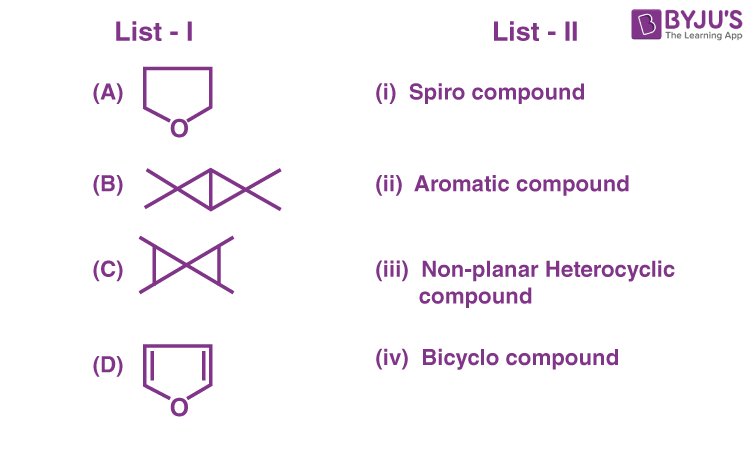(A) (A) – (II), (B) – (I), (C) – (IV), (D) – (III)

(B) (A) – (IV), (B) – (III), (C) – (I), (D) – (II)

(C) (A) – (III), (B) – (IV), (C) – (I), (D) – (II)

(D) (A) – (IV), (B) – (III), (C) – (II), (D) – (I)

Sol.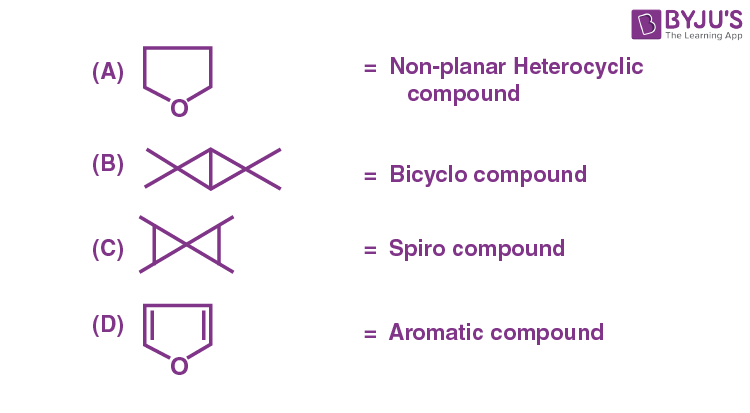13. Choose the correct option for the following reactions.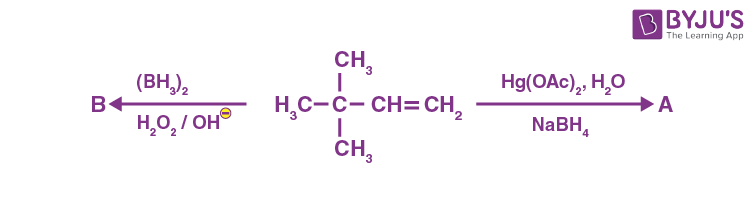(A) ‘A’ and ‘B’ are both Markovnikov addition products

(B) ‘A’ is Markovnikov product and ‘B’ is anti-Markovnikov product

(C) ‘A’ and ‘B’ are both anti-Markovnikov products

(D) ‘B’ is Markovnikov and ‘A’ is anti-Markovnikov product

Sol.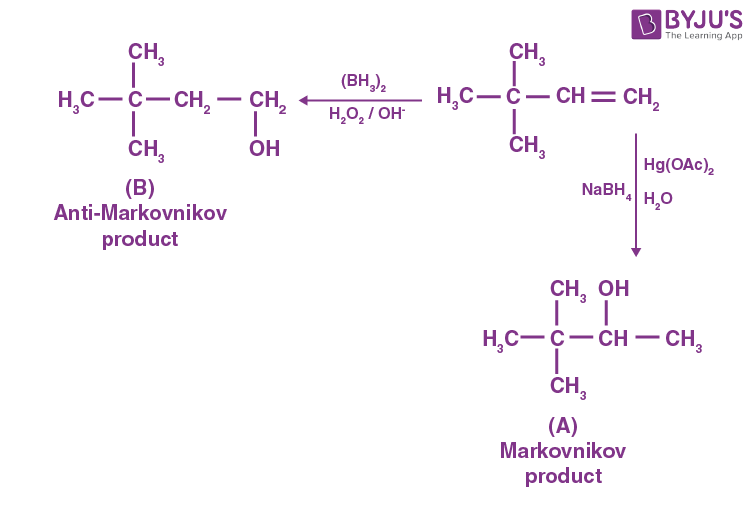14. Among the following marked proton of which compound shows lowest pKa value?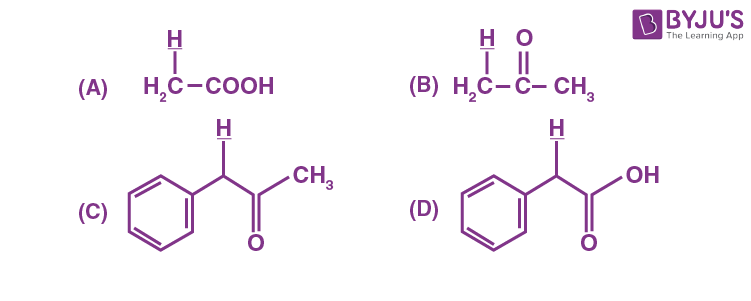Sol.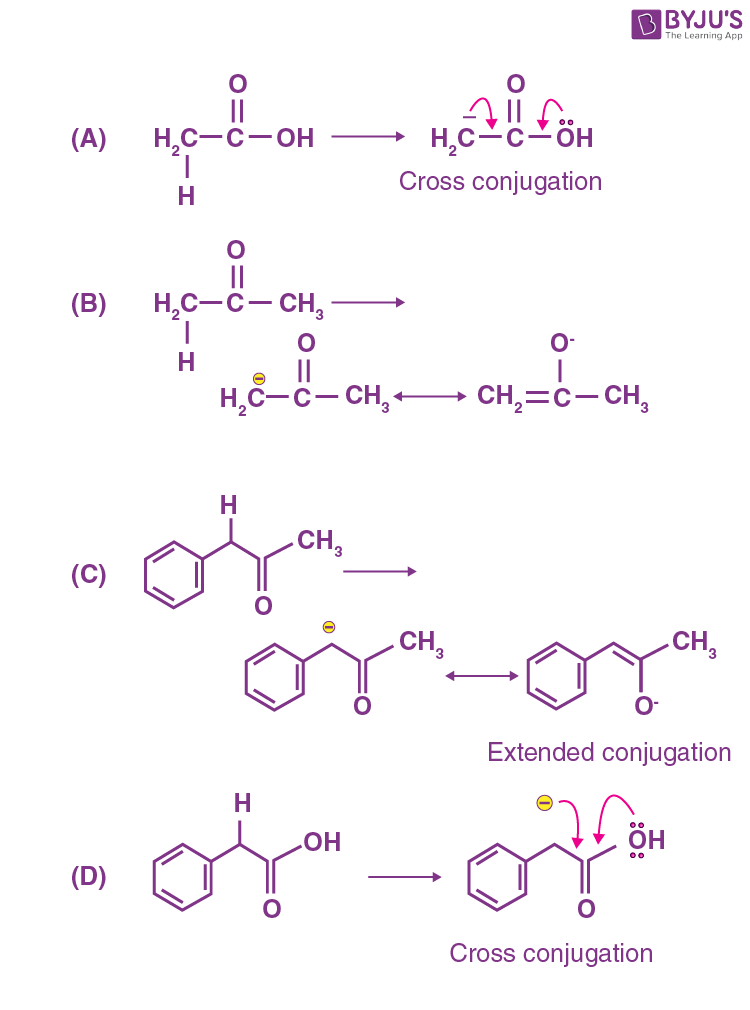The conjugate base of compound (C) is stabilized by extended conjugation. Hence the indicated proton of compound C is most acidic i.e. will have lowest pKa.

15. Identify the major products A and B for the below given reaction sequence.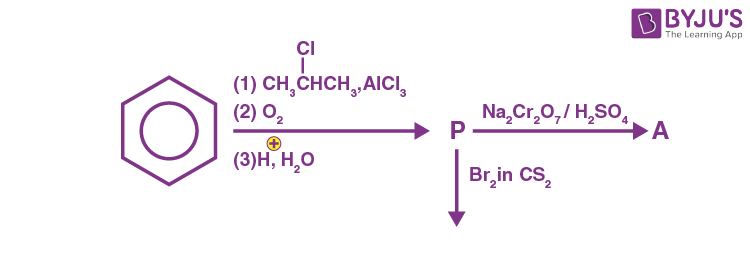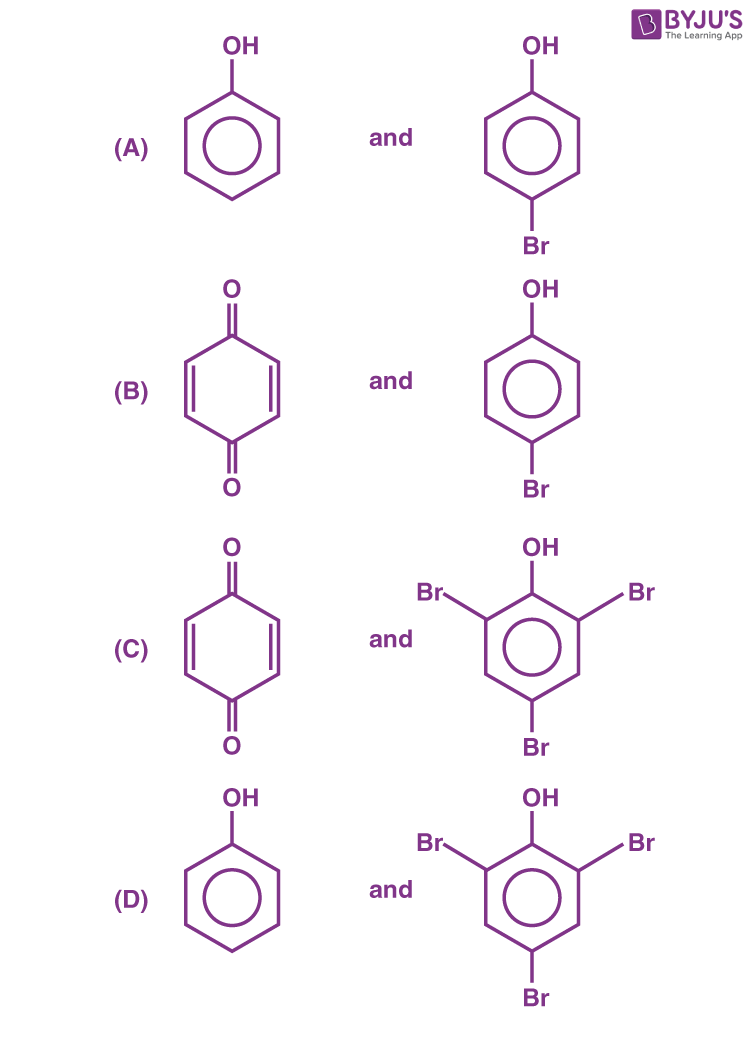Sol.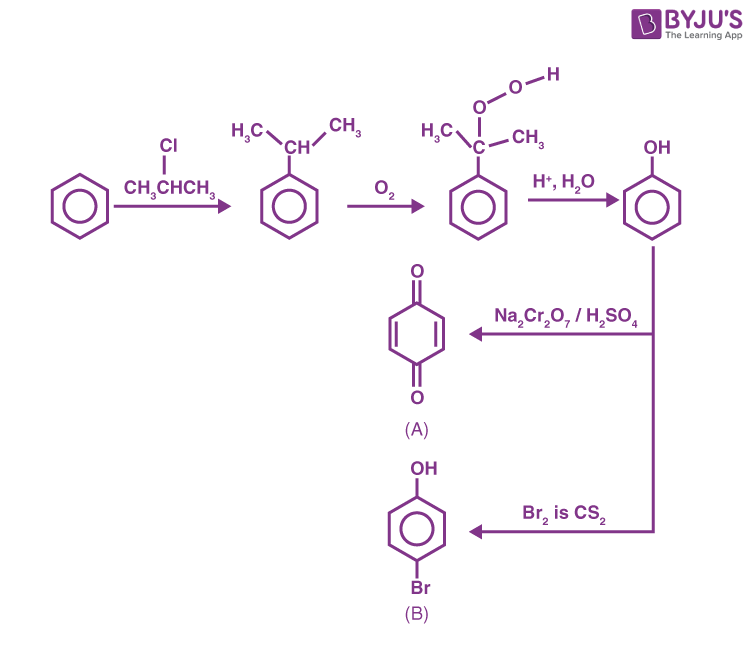16. Identify the correct statement for the below given transformation.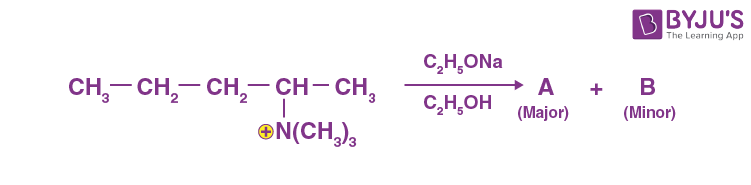$$\begin{array}{l}\left(A\right) A-CH_3CH_2CH=CH–CH_3, B-CH_3CH_2CH_2CH = CH_2, \text{Saytzeff products}\end{array}$$
$$\begin{array}{l}\left(B\right) A-CH_3CH_2CH=CH–CH_3, B-CH_3CH_2CH_2CH = CH_2, \text{Hofmann products}\end{array}$$
$$\begin{array}{l}\left(C\right) A-CH_3CH_2CH_2CH = CH_2, B-CH_3CH_2CH = CHCH_2, \text{Hofmann products} \end{array}$$
$$\begin{array}{l}\left(D\right) A-CH_3CH_2CH_2CH = CH_2,B-CH_3CH_2CH = CHCH_3, \text{Saytzeff products}\end{array}$$

Sol.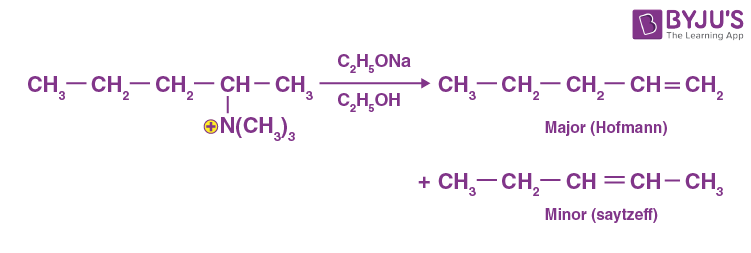17. Terylene polymer is obtained by condensation of:

(A) Ethane-1, 2-diol and Benzene-1, 3 dicarboxylic acid

(B) Propane-1, 2-diol and Benzene-1, 4 dicarboxylic acid

(C) Ethane-1, 2-diol and Benzene-1, 4 dicarboxylic acid

(D) Ethane-1, 2-diol and Benzene-1, 2 dicarboxylic acid

Sol.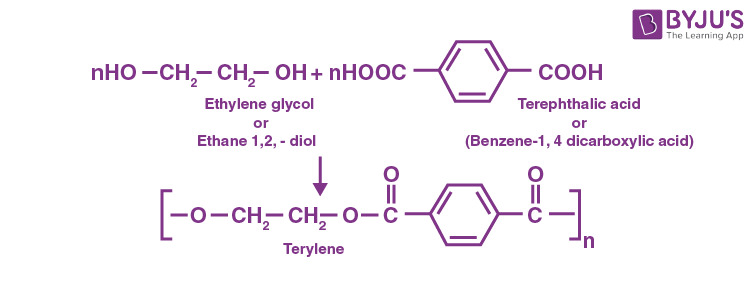18. For the below given cyclic hemiacetal (X), the correct pyranose structure is :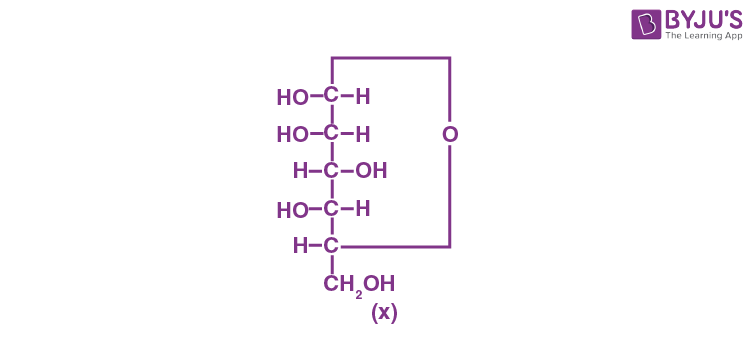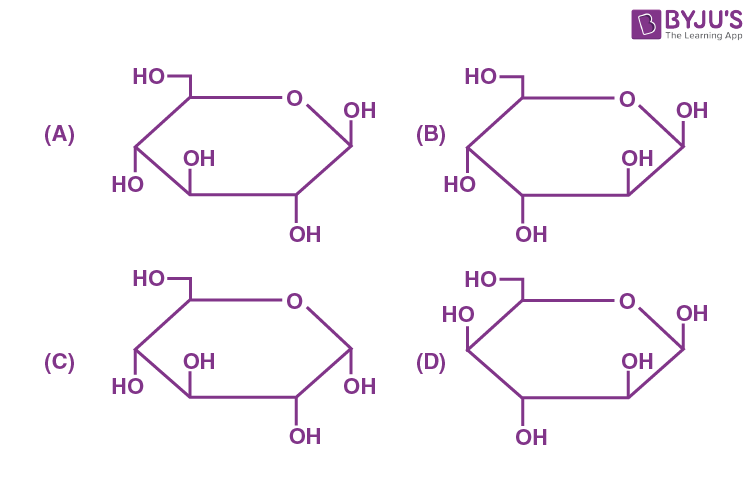Sol.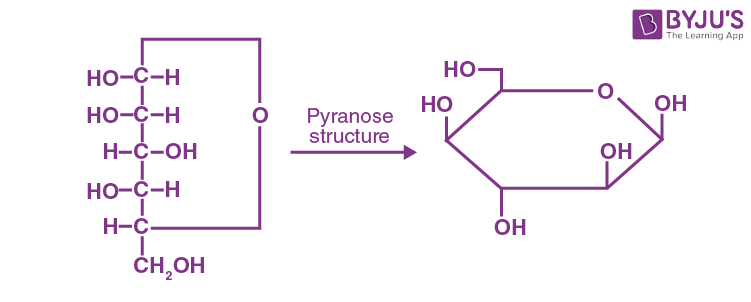–OH on right side will point downwards

–OH on left side will point upwards

19. Statements about Enzyme Inhibitor Drugs are given below :

(A) There are Competitive and Non-competitive inhibitor drugs.

(B) These can bind at the active sites and allosteric sites.

(C) Competitive Drugs are allosteric site blocking drugs.

(D) Non-competitive Drugs are active site blocking drugs.

Choose the correct answer from the options given below:

(A) (A), (D) only

(B) (A), (C) only

(C) (A), (B) only

(D) (A), (B), (C) only

Sol. Drugs can inhibit the attachment of substrate on active site of Enzyme in two ways.

(1) Competitive, (2) Non-competitive

Competitive inhibitors bind on the active site of Enzymes. Non-Competitive inhibitors bind on allosteric site.

20. For kinetic study of the reaction of iodide ion with H2O2 at room temperature :

(A) Always use freshly prepared starch solution.

(B) Always keep the concentration of sodium thiosulphate solution less than that of Kl solution.

(C) Record the time immediately after the appearance of blue colour.

(D) Record the time immediately before the appearance of blue colour.

(E) Always keep the concentration of sodium thiosulphate solution more than that of Kl solution.

Choose the correct answer from the options given below :

(A) (A), (B), (C) only

(B) (A), (D), (E) only

(C) (D), (E) only

(D) (A), (B), (E) only

Sol. To minimize contamination, use freshly prepared starch solution to determine end point. As KI is used in excess to consume all the H2O2 the concentration of sodium thiosulphate solution is less than KI solution. After appearance of blue colour record the time immediately.

SECTION – B

Numerical Value Type Questions: This section contains 10 questions. In Section B, attempt any five questions out of 10. The answer to each question is a NUMERICAL VALUE. For each question, enter the correct numerical value (in decimal notation, truncated/rounded-off to the second decimal place; e.g. 06.25, 07.00, –00.33, –00.30, 30.27, –27.30) using the mouse and the on-screen virtual numeric keypad in the place designated to enter the answer.

1. In the given reaction,

$$\begin{array}{l}X+Y+3Z\rightleftharpoons XYZ_3\end{array}$$

if one mole of each of X and Y with 0.05 mol of Z gives compound XYZ3. (Given : Atomic masses of X, Y and Z are 10, 20 and 30 amu, respectively.) the yield of XYZ3 is _______ g. (Nearest integer)

Sol.

$$\begin{array}{l}\underset{n_{\text{moles}}=1}{X}+\underset{1}{Y}+\underset{0.05}{3Z}\rightleftharpoons xyz_3\end{array}$$

Limiting reagent is

$$\begin{array}{l}Z=\frac{0.05}{3}=0.016\end{array}$$

3 moles of Z → 1 mole of XYZ3

$$\begin{array}{l}0.05 \text{ mole of }Z\rightarrow\frac{1}{3}\times 0.05 \text{ moles of } XYZ_3 \end{array}$$
M.wt. of XYZ3 = 10 + 20 +90

= 120 amu

$$\begin{array}{l}\text{Wt. of} XYZ_3 = \frac{.05}{3}\times 120= 2 g \end{array}$$

2. An element M crystallises in a body centred cubic unit cell with a cell edge of 300 pm. The density of the element is 6.0 g cm–3. The number of atoms present in 180 g of the element is ______× 1023. (Nearest integer)

Sol.

$$\begin{array}{l}a = 300 ~\text{pm}\\ = 300 \times 10^{-12}~\text{m}\\ = 300 \times 10^{-10} \text{cm.}\end{array}$$
$$\begin{array}{l}= 3 \times 10^{-8}~\text{cm}.\end{array}$$
$$\begin{array}{l}\rho = 6 g/cm^3\\ wt = 180 ~\text{gm}\end{array}$$
$$\begin{array}{l}Volume = a^3 \\= \left(3 \times 10^{-8}\right)^3\end{array}$$

Volume occupied per atom

$$\begin{array}{l}=\frac{27\times 10^{-24}}{2}\end{array}$$
$$\begin{array}{l}\rho = 6~~~~~~~wt = 180 \text{g}\end{array}$$
$$\begin{array}{l}\text{Volume } = \frac{180}{6}=30\end{array}$$
$$\begin{array}{l}\text{Number of atoms} = \frac{30 \times 2}{27 \times 10^{-24}}\end{array}$$
$$\begin{array}{l}=\frac{60}{27}\times 10^{24}= 22.2 \times 10^{23}\end{array}$$

3. The number of paramagnetic species among the following is ______.

$$\begin{array}{l}B_2, Li_2, C_2, C_2^-, O_2^{2-},O_2^+ \text{ and } He_2^+\end{array}$$

Sol. B2 → 10 e paramagnetic

Li2 → 6 e

C2 → 12 e

$$\begin{array}{l}C_2^-\rightarrow 13e^-\ \text{paramagnetic}\end{array}$$
$$\begin{array}{l}O_2^{-2}\rightarrow 18e^-\end{array}$$
$$\begin{array}{l}O_2^{+}\rightarrow 15e^-\ \text{paramagnetic}\end{array}$$
$$\begin{array}{l}He_2^{+}\rightarrow 3e^-\ \text{paramagnetic}\end{array}$$

Species with odd number of electrons are paramagnetic except boron and oxygen.

4. 150 g of acetic acid was contaminated with 10.2 g ascorbic acid (C6H8O6) to lower down its freezing point by (x × 10–1)°C. The value of x is ______. (Nearest integer)

(Given : Kf = 3.9 K kg mol–1; molar mass of ascorbic acid = 176 g mol–1)

Sol. M.wt. of Acetic acid = 60 g

M.wt. of Ascorbic acid = 176 g

$$\begin{array}{l}\Delta T_f = K_f m\end{array}$$
$$\begin{array}{l}\Delta T_f = \frac{3.9 \times 10.2 \times 1000}{176 \times 150}\end{array}$$
$$\begin{array}{l}\Delta T_f =1.506\\= 15.06 × 10^{–1}= 15\end{array}$$

5. Ka for butyric acid (C3H7COOH) is 2 × 10–5. The pH of 0.2 M solution of butyric acid is ______× 10–1. (Nearest integer) (Given log2 = 0.30)

Sol.

$$\begin{array}{l}K_a = C\alpha^2 ~~~~~~~C = 0.2 \text{M}\end{array}$$
$$\begin{array}{l}\alpha= \sqrt{\frac{K_a}{C}}~~~~~~~K_a = 2 \times 10^{-5}\end{array}$$
$$\begin{array}{l}=\sqrt{\frac{2 \times 10^{-5}}{2\times 10^{-1}}}= 10^{–2}\end{array}$$
[H+] = Cα

= 0.2 × 10–2

= 2 × 10–3

pH = 3 – log2

= 3 – 0.30

= 2.7

pH = 27 × 10–1

6. For the given first order reaction A → B, the half-life of the reaction is 0.3010 min. The ratio of the initial concentration of reactant to the concentration of reactant at time 2.0 min will be equal to ______. (Nearest integer)

Sol.

$$\begin{array}{l}t_{1/2}=\frac{0.693}{K}~~~~~~~t_{1/2} \text{ given }= 0.3010\end{array}$$
$$\begin{array}{l}K = \frac{0.693}{0.3010} \\K = 2.30 \end{array}$$
$$\begin{array}{l}K = \frac{2.303}{t}\log \frac{(A_0)}{(A_t)}\end{array}$$

A0 → initial concentration of reactant

At → concentration of reactant at time t

$$\begin{array}{l}2.303 = \frac{2.303}{2}\log \frac{(A_0)}{(A_t)}\end{array}$$
$$\begin{array}{l}2=\log \frac{(A_0)}{(A_t)}\end{array}$$
$$\begin{array}{l}100=\frac{(A_0)}{(A_t)}\end{array}$$

7. The number of interhalogens from the following having square pyramidal structure is :

CIF3, IF7, BrF5, BrF3, I2CI6, IF5, CIF, CIF5

Sol. ClF3 → 3 σ bond + 2 lone pair

IF7 → 7 σ bond + 0 lone pair

BrF5 → 5 σ bond + 1 lone pair → Square pyramidal

BrF3 → 3 σ bond + 2 lone pair

I2Cl6 → 4 σ bond + 2 lone pair

IF5 → 5 σ bond + 1 lone pair → Square pyramidal

ClF → 1 σ bond + 3 lone pair

ClF5 → 5 σ bond + 1 lone pair → Square pyramidal

8. The disproportionation of MnO42- in acidic medium resulted in the formation of two manganese compounds A and B. If the oxidation state of Mn in B is smaller than that of A, then the spin-only magnetic moment (μ) value of B in BM is _______. (Nearest integer)

Sol.

$$\begin{array}{l}\overset{+6}{3Mn}O_4^{-2}+4H^+\rightarrow \overset{+4}{Mn}O_2 + \overset{+7}{Mn}O_4^-\end{array}$$

Mn → 4s23d5

Mn+4 → 3d3

n = 3

$$\begin{array}{l}\mu = \sqrt{n(n+2)}\end{array}$$
$$\begin{array}{l}=\sqrt{3(5)}\end{array}$$
$$\begin{array}{l}=\sqrt{15} = 3.87 \approx 4 ~\text{B.M.}\end{array}$$

9. Total number of relatively more stable isomer(s) possible for octahedral complex [Cu(en)2(SCN)2] wiil be ______.

Sol. [Cu(en)2(SCN)2]

Total isomers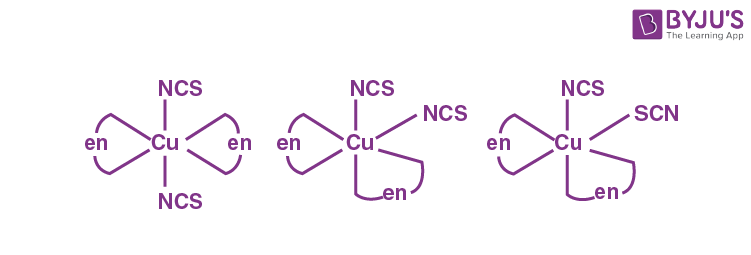10. On complete combustion of 0.492 g of an organic compound containing C, H and O, 0.7938 g of CO2 and 0.4428 g of H2O was produced. The % composition of oxygen in the compound is _______.

Sol.

$$\begin{array}{l}\% \text{ of } H = \frac{2}{18}\times \frac{\text{Wt. of } H_2O}{\text{wt. of organic compound}}\times 100\end{array}$$
$$\begin{array}{l}=\frac{2}{18}\times \frac{0.4428}{0.492}\times 100\end{array}$$

= 0.11 × 0.9 × 100

= .099 × 100 = 9.9

$$\begin{array}{l}\% \text{ of }C = \frac{12}{44}\times \frac{0.7938}{0.492}\times 100\end{array}$$

= 0.27 × 1.61 × 100

= 43.47

% Oxygen = 100 – (43.47 + 9.9)

= 100 – 53.37 ≃ 46

### Download PDF of JEE Main 2022 July 28 Shift 1 Chemistry Paper & Solutions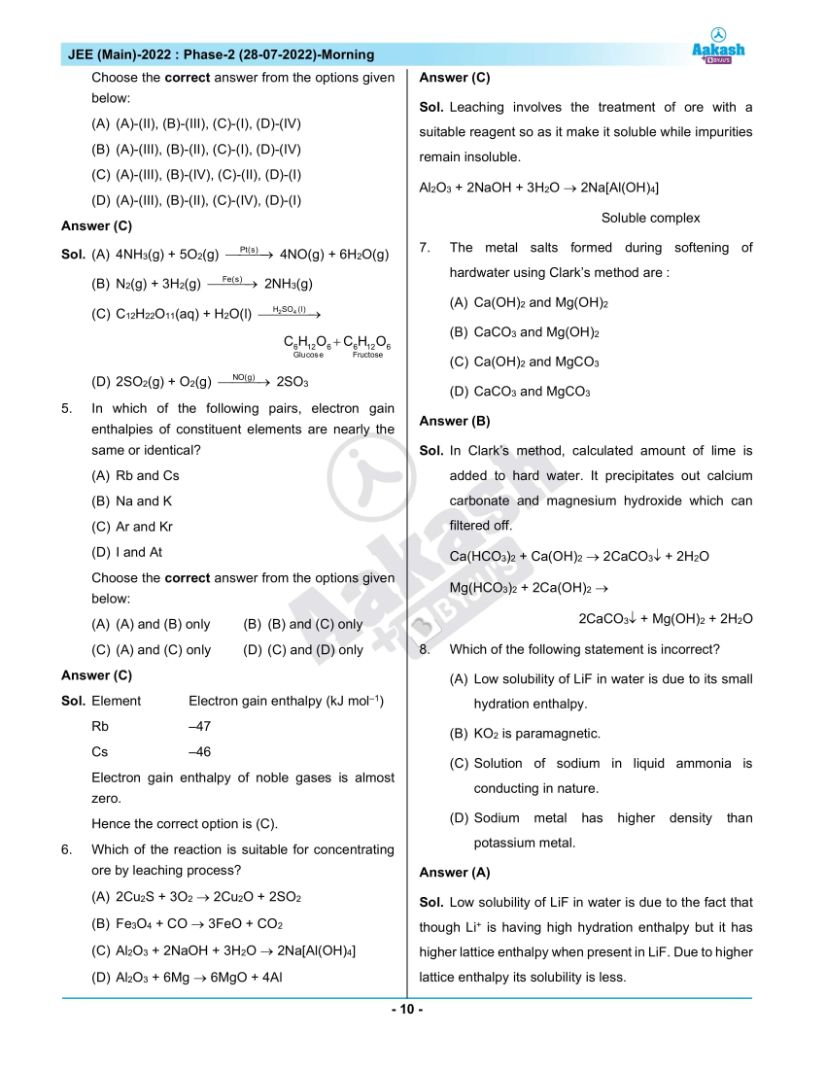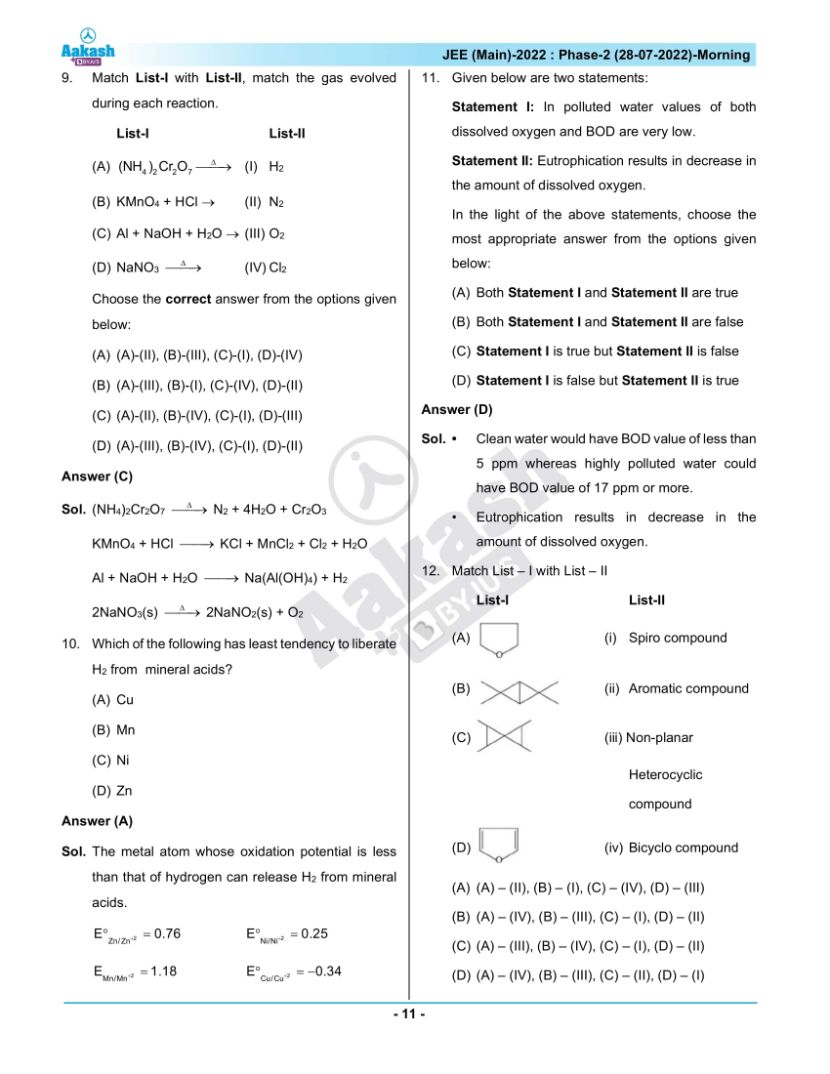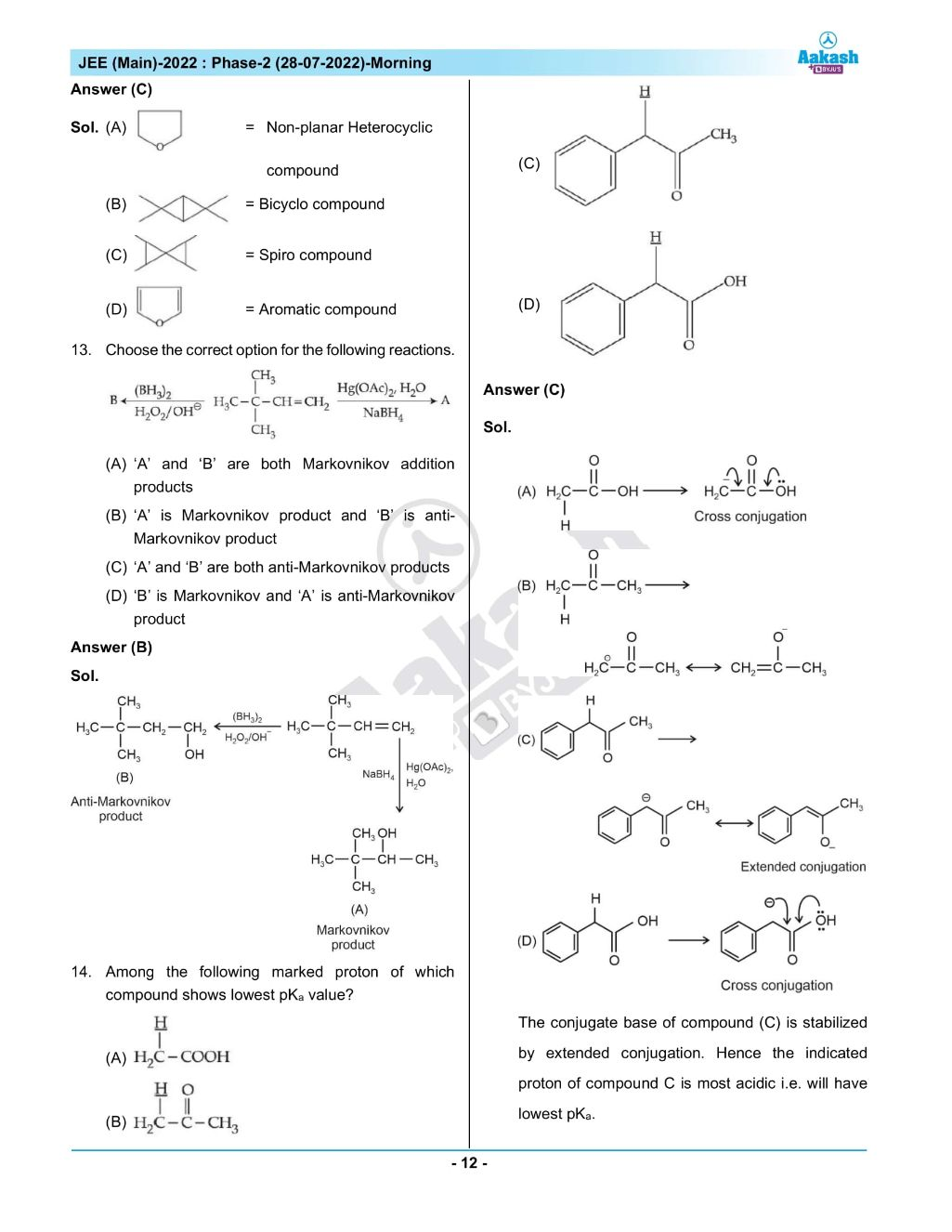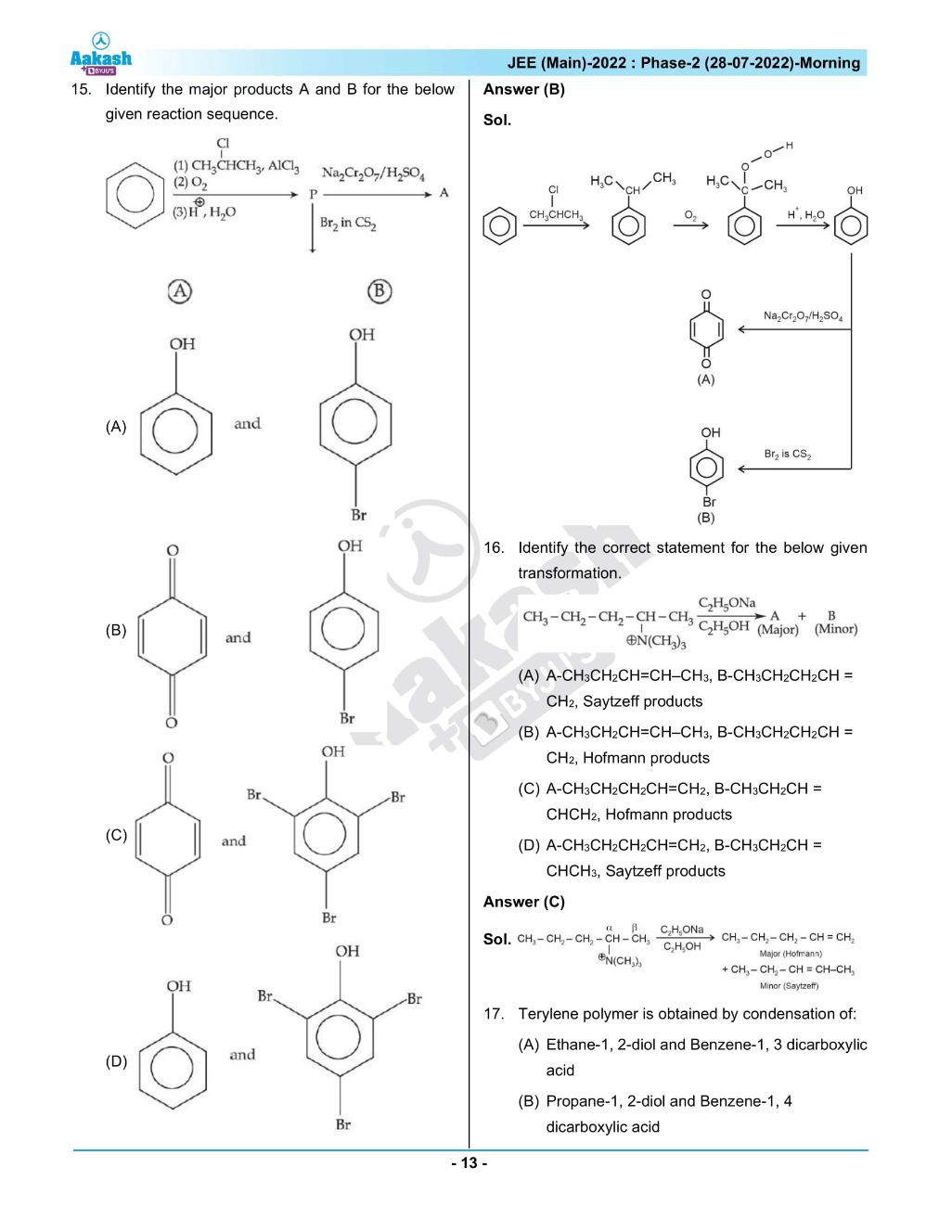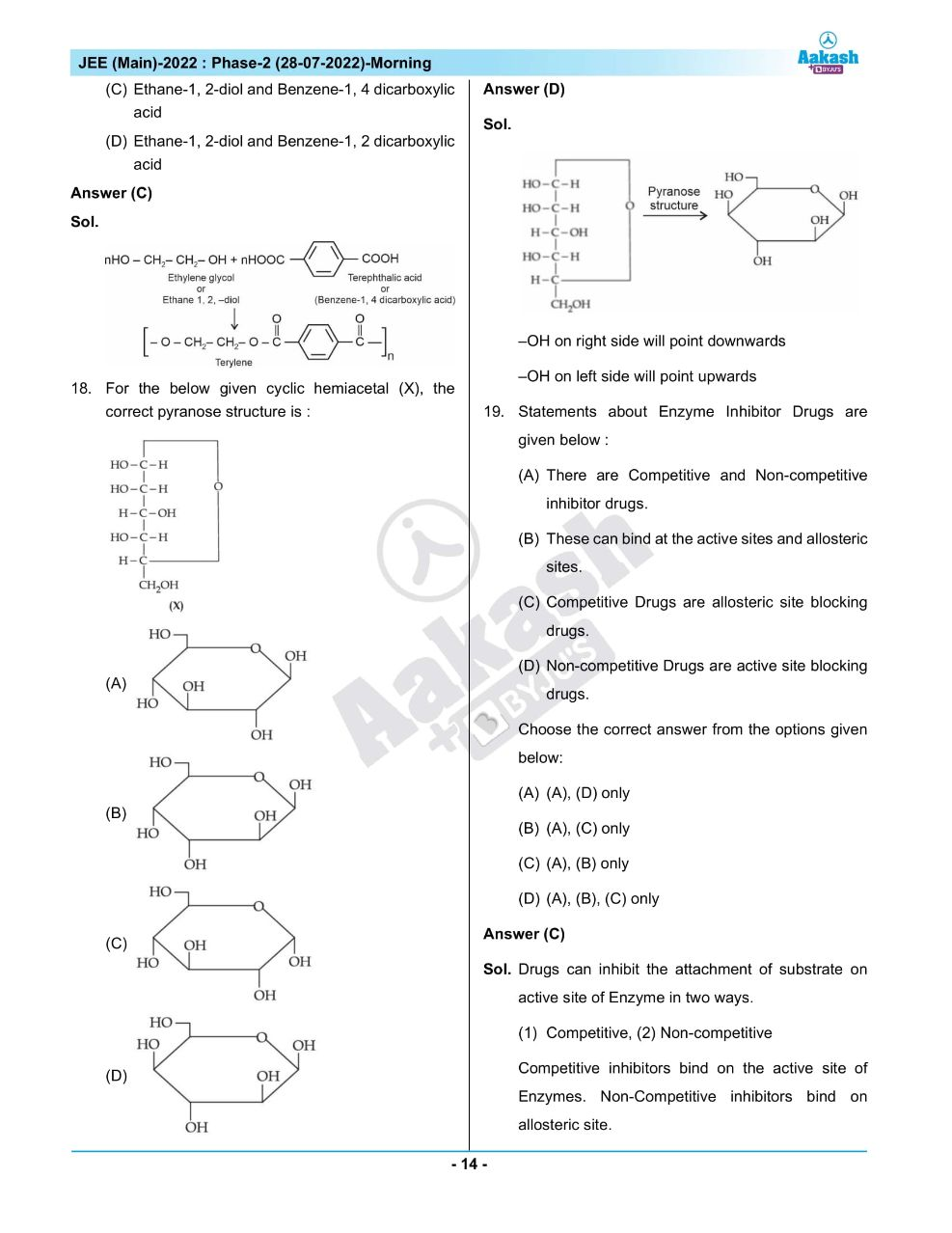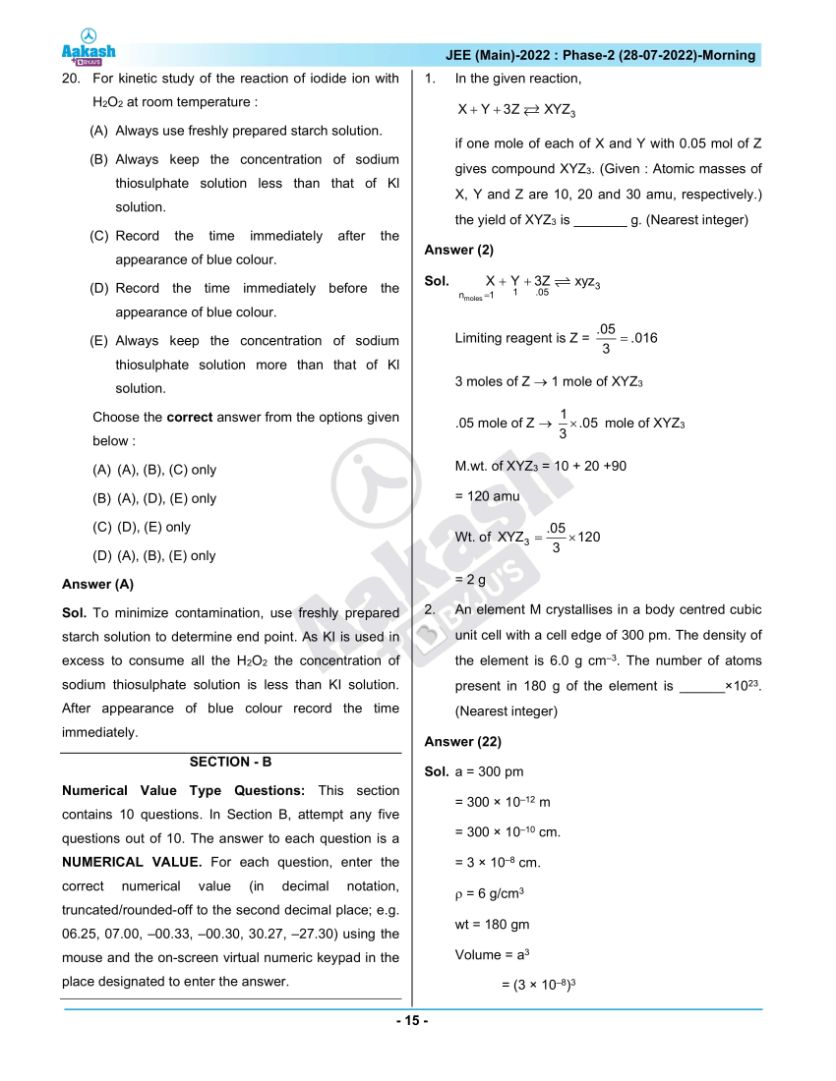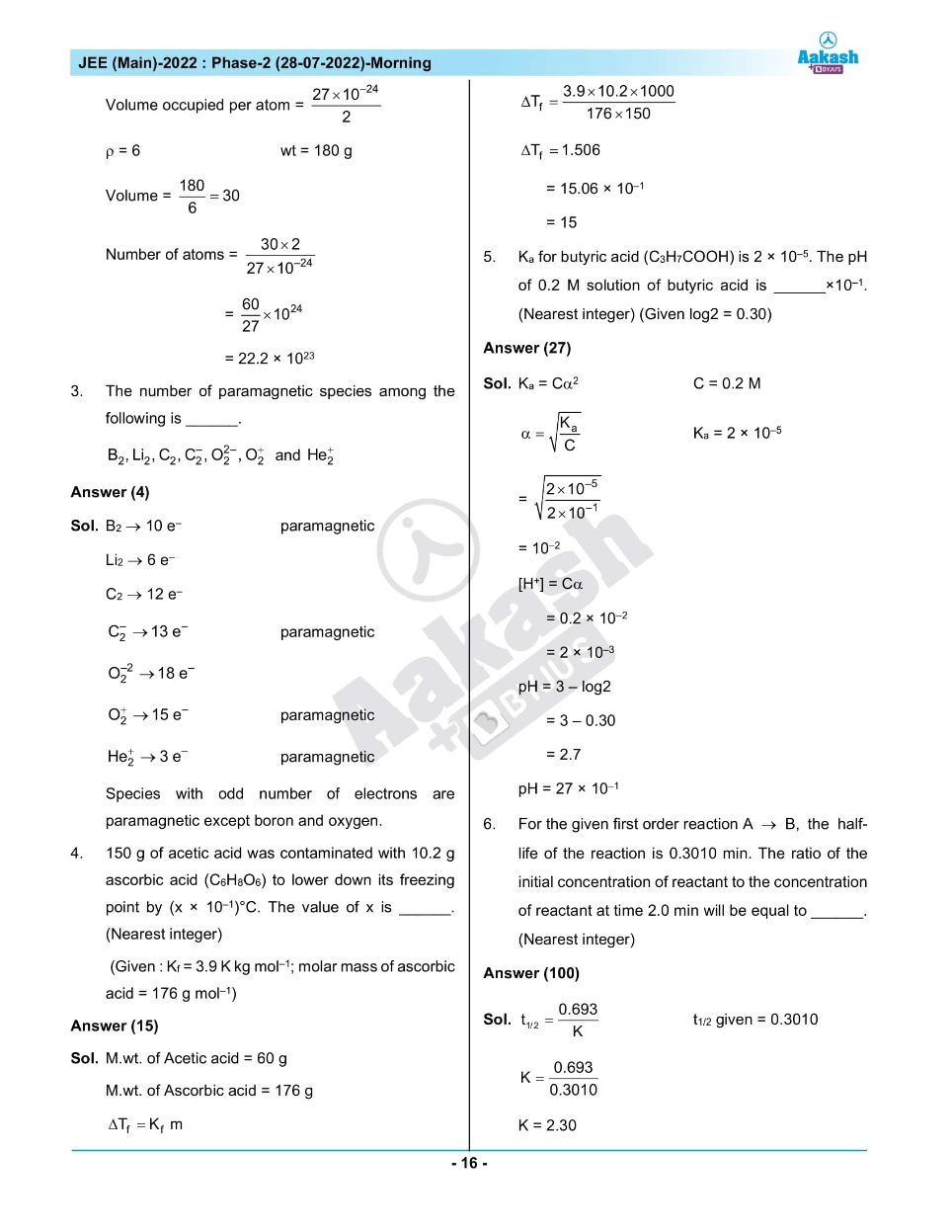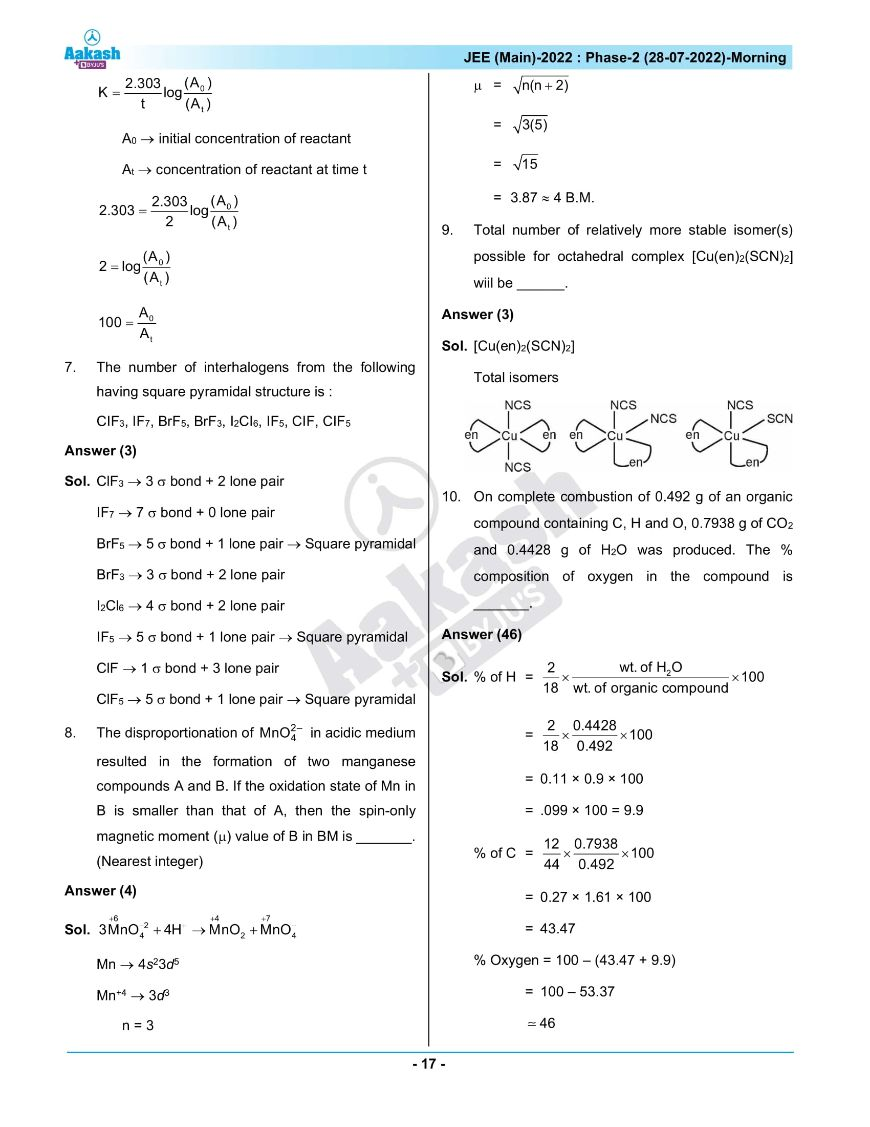## JEE Main 2022 July 28th Shift 1 Paper Analysis